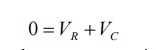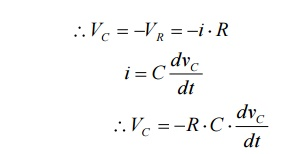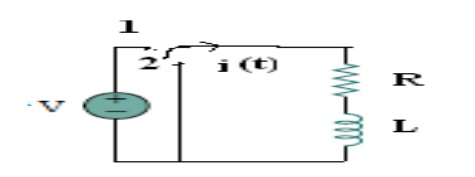Home | | Circuit Theory | Analysis of undriven(source free) series RC and RL circuits

# Analysis of undriven(source free) series RC and RL circuits

1) Explain the analysis of undriven series RL circuits. Or Explain the analysis of source free series RL circuits. 2)Explain about discharging of capacitor through resistor in source free series RC circuit. Or Explain the analysis of undriven or source free series RC circuits.

Discharging of capacitor through resistor in source free series RC circuit. Or Analysis of undriven or source free series RC circuits.Consider network shown in fig. the switch k is moved from position 1 to 2 at reference time t = 0.

Now before switching take place, the capacitor C is fully charged to V volts and it discharges through resistance R. As time passes, charge and hence voltage across capacitor i.e. Vc decreases gradually and hence discharge current also decreases gradually from maximum to zero exponentially.

After switching has taken place, applying kirchoff’s voltage law,Where VR is voltage across resistor and VC is voltage across capacitor.Above equation is linear, homogenous first order differential equation. Hence rearranging we have,The above expression is nothing but discharge current of capacitor. The variation of this current with respect to time is shown in fig.

This shows that the current is exponentially decaying. At point P on the graph. The current value is (0.368) times its maximum value. The characteristics of decay are determined by values R and C, which are 2 parameters of network.

For this network, after the instant t = 0, there is no driving voltage source in circuit, hence it is called undriven RC circuit.Analysis of undriven series RL circuits (or) Analysis of source free series RL circuits

Current decay in source free series RL circuit: -At t = 0- , switch k is kept at position ‘a’ for very long time. Thus, the network is in steady state. Initial current through inductor is given as,Because current through inductor can not change instantaneously

Assume that at t = 0 switch k is moved to position ‘b’,From the graph, H is clear that current is exponentially decaying. At point P on graph. The current value is (0.363) times its maximum value. The characteristics of decay are determined by values R and L which are two parameters of network.

The voltage across inductor is given byStudy Material, Lecturing Notes, Assignment, Reference, Wiki description explanation, brief detail
: Analysis of undriven(source free) series RC and RL circuits |